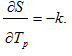# ufpGetJac()

Get the pointer to the source Jacobian.

## Syntax

jac = ufpGetJac ( ufpHd ) ;

## Type

AcuTrace User-Defined Function Particle Routine

## Parameters

ufpHd (pointer)
The opaque handle which was passed to the user function.

## Return Value

The return value is a pointer to a one-dimensional array. Once the pointer is obtained, you should fill it with the values of the Jacobian with respect to the user function variables. The size of the Jacobian is square of the number of user equation variables.

If the source term does not depend on the user function variable, the Jacobian is identically 0 and does not need to be set.

It is your responsibility to determine the correct formulation of the Jacobian.

## Description

This routine returns the pointer to the source Jacobian.

A user defined function providing the source term for heat transfer between the fluid and the particle could be written as:
Void usrTemp (
UfpHd   ufpHd,
Real*   outVec,
Integer nItems,
Integer vecDim
)
{
Real* jac ;
Real* usrVals ;
Real* t_fluid ;
Real* t_particle ;
Real  cond ;
...
usrVals           = ufpGetUsrVals (  ufpHd                                  ) ;
jac               = ufpGetJac     (  ufpHd, UFP_JAC_UDF_VARIABLES           ) ;
t_fluid           = ufpGetFlowData(  ufpHd,UFP_FLOW_TEMPERATURE             ) ;
t_particle        = ufpGetUdfData (  ufpHd,0                                ) ;
cond              = usrVals;
outVec[0)         = cond * ( t_fluid – t_particle

/*---------------------------------------------------------------------------
* The jacobian is the derivative of the source with respect to the particle
* temperature
*---------------------------------------------------------------------------
*/
jac            = -cond ;
...
}
In this case, the evolution equation is(1)where ${T}_{p}$ is the particle temperature, ${T}_{f}$ the fluid temperature, and $k$ the conductivity. The Jacobian is(2)## Errors

This routine expects a valid ufpHd as an argument; an invalid arguments returns an error.